# One try | QC Explained

## Phase kickback and Bernstein-Vaz algorithm

This one is technical. Feeling rusty on your quantum computing fundamentals? Don’t read it. Save it. Bookmark this article for later when you’ve brushed up. You’ll be glad you did, especially if you’re a beginner in QC and trying to understand phase kickback.

I’m thinking of a number in binary.

If you wanted to guess my number, it could take as many guesses as there are possibilities. If it was `101101`, it would take you upwards of 64 tries (2⁶)!

Now, if you ask our everyday computers to guess that number, the number of tries needed to guess it would be reduced to the number of digits. So if I chose `101101` again, it would take 6 tries (if you’re interested in why, check out this video). Not bad right?

Sounds crazy right? But that’s what this magical quantum algorithm can do. It’s the Bernstein-Vazirani algorithm and in this article, I’ll show you how it works.

To get started, we need to understand the backbone of the whole algorithm: phase kickback. Once you get it, the algorithm itself will be a cinch.

If your head spins at the mention of relative phase or superposition, I’d highly recommend giving this a read, it covers these concepts extensively.

# An “eigenvector-free” explanation to phase kickback

## Controlled NOT quantum gate

To get us all on the same page, let’s debrief the quantum gate at the heart of phase kickback: CNOT. The controlled not gate (CNOT) is a two-qubit gate that performs a bit flip on the second qubit (referred to as the target qubit) under the condition that the first qubit (referred to as the control qubit) is 1. If the control qubit is in the state 0, then it leaves the target qubit (target qubit) unchanged. Here’s the circuit representation of this: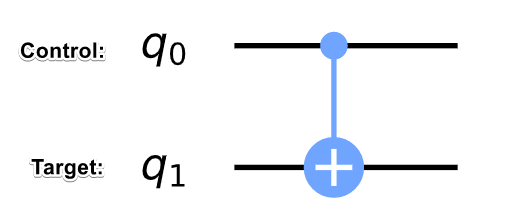Circuit representation of CNOT: q0 as the control, and q1 as the target

And here’s the CNOT being applied to each computational basis state: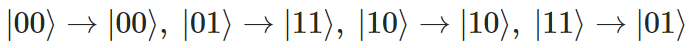On notation, q1 comes first followed by q0 (this notation will apply for the rest of the article)

## A better way to think of the CNOT

Mathematically speaking, we would say that the amplitudes for the states `|01⟩`and `|11⟩` were swapped (refer above) but that doesn’t uphold when trying to explain the physical operation, and that’s one key distinction that must be made. Often, we get lost in our hairy math expressions and forget that tools like linear algebra — albeit powerful — are just that, tools. It helps us abstract quantum weirdness into coherent expressions but at the end of the day, logic gates like the CNOT are physical processes and thus we should think of them like so.

I ask you to forget the matrices for a moment and actually imagine the quantum state as it’s being affected by the CNOT. As the two qubits travel through time, if it’s in the state `|01⟩` it will become `|11⟩` — taking all of its properties with it, including the amplitude.

You can think of it as a person moving into a new house. He/she evolves into a new state but still carries most of the properties from beforehand like hair, skin colour, etc. Looking at it from the perspective of time evolution differs physically from thinking of it as swapping states. Keep this in mind when we tackle phase kickback.

## So what is it?

If I give you the textbook definition right now, it’ll breeze right over your head so let’s build towards it with two different examples. The first one will be a meaningless application of phase kickback, but it’ll help us understand the necessary conditions for phase kickback to have a true effect. The second circuit we build will add one feature from the prior one that’ll completely change the circuit outcome.

## When phase kickback happens, but it doesn’t matter

Let’s build our first circuit. We put the “control qubit” in the state `|1⟩` using an X gate and then apply an X gate followed by a Hadamard to bring the state of the “target qubit” to `H(X|0⟩)` . Here’s what the state and circuit look like so far:

Next, we apply a CNOT gate…

Notice that in the case that an X gate is applied to `q1` (as a part of the CNOT), the state vector for `q1` is already on the x-axis (I encourage you to check by plotting it on the Bloch sphere), hence it won’t affect `q1`. That’s weird, isn’t it? I just told you that the irrespective of the control qubit’s state! Let’s go step by step: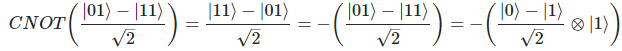Notice how the last step has a negative sign in front of the whole quantum state, this can be disregarded as a global phase. If you have no idea what that is, check the last section of this article out.

Wow, not only was `q1` untouched but applying the CNOT to our quantum state has done nothing since we just modified the global phase! To drill this home, the two quantum states below are physically equivalent.

So yes, the CNOT hasn’t actually changed our quantum state but there’s something even more important to notice, `-|11⟩` became `-|01⟩` , and `|01⟩` evolved to `|11⟩` , this shows that the `q1``q0` Here’s the same proof as above but with a detailed step indicating the phase kickback on `q0`.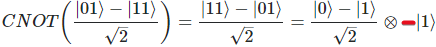Notice how this is the exact same quantum state as before except there’s now a negative phase on |1⟩ (q0) but as mentioned above this can be factored out to result in the same quantum state we started with.

In this case, the phase kickback had no effect since the second qubit was in a computational basis state, However, this isn’t always the case, so let’s look at an example where this phenomenon actually matters.

## The key differentiator

Our second circuit will be similar to our last one, but with one key difference. Instead of applying an X gate on `q0`, we’re going to apply a Hadamard. Let’s see what the circuit looks like.

I inserted a barrier between the Hadamard’s and the CNOT so that we could examine the quantum state before and after the CNOT. Let’s see what the state vector is before applying the CNOT.

Okay, so what’s going to happen when we apply the CNOT? Well, we know that the CNOT gate evolves the state `|01⟩` to `|11⟩` and vice versa. In our circuit, we observe the two basis states `|01⟩` and `-|11⟩` , hence it would be reasonable to place your bets on `|01⟩ → |11⟩ ; -|11⟩ → -|01⟩` . Let’s see if we’re correct.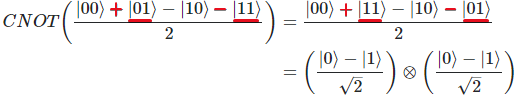Applying the CNOT on q1 has kicked the relative phase of q1 up to q0

Hurrah! We were right! This is a prime example where phase kickback actually matters. Observe that `q0` didn’t have a relative phase before the CNOT, but after applying the gate we can see that it has a relative phase of `-1`. What happened? Not only was the target qubit unaffected but it also kicked its relative phase to `q0`.

## On control and target

I’ve been using the terms control and target often in this article but to be honest, this isn’t completely correct. Calling `q0` a control qubit implies that it sits on a pedestal with sweeping control of `q1` but that’s simply not the case. As demonstrated with phase kickback, `q0` can often be affected. A better way to think of the CNOT is a physical operation that transforms `|01⟩` into `|11⟩` and vice versa.

So under that lens, it makes sense that `q0` absorbed a relative phase. The CNOT transforms the state `|11⟩` to `|01⟩` so if there’s an accompanying phase that’s with `|11⟩` , it’ll naturally get carried on to the new basis state:`-|11⟩ --> -|01⟩` .

To avoid the muddy connotation, I’ll refer to the control qubit as the ancilla qubit.

We now understand phase kickback in the context of a CNOT but this phenomenon occurs in other situations as well.

## Time for a generalized definition

It’s bullet time:

• As you know, all quantum logic gates are unitary and can be thought of as rotations on the Bloch sphere
• Applying a unitary operation on an eigenstate just means that you’re applying a rotation on a state vector that is on the axis you’re rotating around. Naturally, the rotation doesn’t physically affect the state vector, .
• For example, if I apply an X gate while the state is on a Hadamard basis (on the x-axis), then nothing will happen since the X gate performs a π rotation around the x-axis. However, a global phase will be added to the state such that it’s now in `e^iπ|+⟩` or `-|+⟩`.

When you apply a controlled operation on a target qubit that is in an eigenstate of the unitary, what’s essentially happening is that you’re tacking on a global phase to that target qubit.

Normally, this wouldn’t matter but since it’s a controlled operation, the target qubit will be in a superposition of having incurred this global phase, and not having incurred it. Thus, a relative phase is born between the quantum states (including the ancilla) that have the global phase and those that do not.

This phenomenon is what we refer to as phase kickback.

## Why a controlled operation?

• If we were to not use a controlled operation, it would target all states and thus we would just incur a global phase.

# The easy part — Bernstein Vazirani algorithm

Now that we get what phase kickback is, we can guess any number in one try using the Bernstein Vazirani algorithm.

## Step 1: Initialize qubits to 0

After you know what your binary number is, initialize the qubits to `|0⟩` .

How may qubits though?

In general, you’ll need as many qubits as there are binary digits in your number plus an extra one. Let’s take the example of `1010` to create this circuit. We’ll need 5 qubits (4+1) for this example.

To access all possible states in superposition, we’re going to apply a Hadamard to every qubit. For the last qubit, however, we will apply an X gate before the Hadamard such that the qubit has a negative relative phase. I’ll explain the use of this last crucial qubit in the following step.

Since the Hadamard gate is unitary, applying it twice reverts the transformation imposed by the first Hadamard gate. If there’s nothing between the two Hadamard gates, it will return back to `|0⟩` . But the point in the Hadamard sandwich is to tactfully place certain gates in the sandwich such that it tells us something about the state. More of this in the next step.

## Step 4: Filling the sandwich with an oracle

What goes in between the Hadamard sandwich is crucial, and this middle part is the oracle. The job of the oracle is to discern between the correct number and the number we input, in this case, we’re inputting all possible numbers in a superposition.

How can we distinguish the correct number? Well, if you recall what we learned with phase kickback we can leverage the fact that `q4` has a negative phase to strategically dish out this phase to other qubits using CNOT. If we set the target qubit to `q4` and connect a CNOT for each digit that is 1 in the binary number, then we can kick a negative phase to each of those qubits. The point of `q4` is simply to dish out negative relative phases to each qubit representing a 1 through the use of CNOTs. This differentiates the “1” qubits from the rest. The last line of Hadamard's will then transform the “1” qubits to `|1⟩` and the rest to `|0⟩` ; thereby giving us our random number.

Recall that each qubit except the last one represents a digit in the binary number we chose. So for every qubit that represents a digit that is 1, we’re going to set those qubits as the controls for a CNOT targeted at `q4` . This will do nothing to `q4` but it will kick negative relative phases back to each qubit representing a binary digit that is 1. So since our number is `1010` , our circuit would look like this:

As you might have noticed, we have encoded the oracle such that the circuit performs a bit flip on any qubit that is supposed to represent a 1 in the binary number.

Don’t we need to know the answer to make the oracle? Feel like cheating…

Using the oracle might seem discomforting at first because we’re technically using the solution data to make it but there is no other way to discern between the input and the solution. Even the classical computer’s black box is aware of the answer but it still takes much longer to solve it.

In these trivial examples, we’ll know the solution beforehand but as we progress in quantum computing the oracle will be made such that it can discern between the solution and the input; you don’t need to know the solution to do that.

## Step 5: Measurement

After measuring all of the qubits except for the last one we can arrive at our random number in one try!

Unsurprisingly, here are the counts:

As promised, we got `1010` as the result of the circuit!

# Recap

That was a lot so here’s the bullet recap:

• To remember phase kickback, think back to the CNOT example. A basis state with a negative phase switches with another basis state such that the ancilla qubit now has the negative relative phase as well.
• The Bernstein Vazirani algorithm leverages phase kickback to mark all of the binary digits that are 1 to allow us to measure the whole number in one try.

--

--